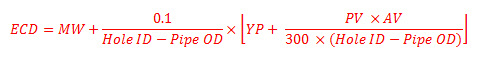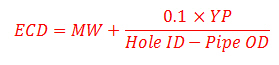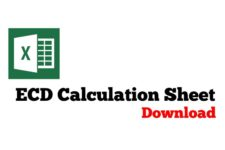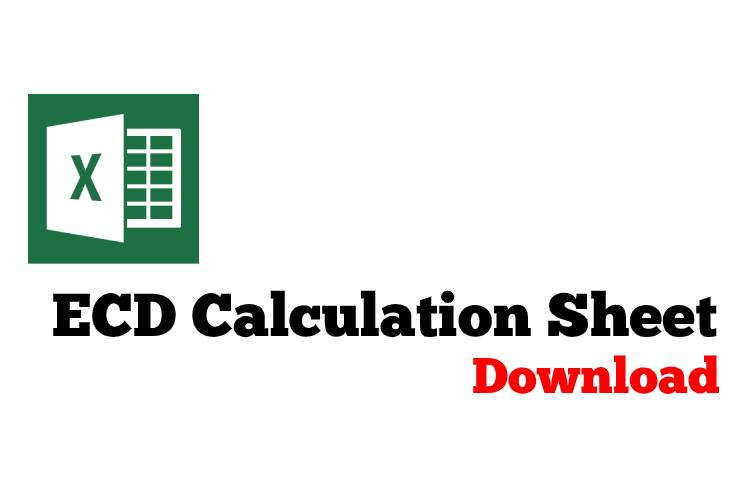## Equivalent Circulating Density (ECD) Using Yield Point for MW More than 13 ppg

Another equation to determine equivalent circulating density is to use yield point.This formula below is used to calculate the ECD and it is good for mud weight more than to 13.0 ppgWhere:

ECD is equivalent circulating density in ppg.

MW is mud weight in ppg.

Hold ID is inside diameter of hole in inch.

Pipe OD is outside diameter of pipe in inch.

YP is mud yield point.

YP can be calculated by these following equations.

YP = Reading at 300 rpm – PV

AV is annular velocity in ft/min

AV can be determined by the following equation.

AV in ft/min = (24.5 x Q) ÷ (Dh2 – Dp2)

where

Q = flow rate in gpm

Dh = inside diameter of casing or hole size in inch

Dp = outside diameter of drill pipe, drill collars, or tubing in inch

## Equivalent Circulating Density (ECD) Using Yield Point for MW less than 13 ppg

Another way to determine equivalent circulating density (ECD) is to use yield point.This formula below is used to calculate the ECD and it is good for mud weight less than or equal to 13.0 ppg.Where:

ECD is equivalent circulating density in ppg

MW is mud weight in ppg

Hold ID is inside diameter of hole in inch

Pipe OD is outside diameter of pipe in inch

YP is mud yield point

YP can be calculated by these following equations

YP = Reading at 300 rpm – PV

## Equivalent Circulating Density (ECD) in both Oilfield and Metric UnitEquivalent Circulating Density (ECD) is the effective density that combines current mud density and annular pressure drop. ECD is vital for drilling engineering since it limits how depth of each section to be drilled and leads to losses.The equivalent circulating density formula is shown below;

## Oilfield Unit

Equivalent Circulating Density (ECD) = (Annular Pressure Loss ÷ 0.052 ÷ True Vertical Depth (TVD)) + (Current Mud Weight)

Equivalent Circulating Density (ECD) in ppg

Annular Pressure Loss in psi

True Vertical Depth (TVD) in ft

Current Mud Weight in pppg

Example:

Annular pressure loss = 400 psi
True Vertical Depth = 8,000 ft
Current mud weight in ppg = 10 ppg

ECD in ppg =   (400 psi ÷ 0.052 ÷ 8,000 ft) +10.0 ppg

ECD = 11.0 ppg

## Metric Unit

Equivalent Circulating Density (ECD) = (Annular Pressure Loss × 1000 ÷ 9.81÷ True Vertical Depth (TVD) ) + (Current Mud Weight)

Equivalent Circulating Density (ECD) in kg/m3

Annular Pressure Loss in KPa

True Vertical Depth (TVD) in m

Current Mud Weight in kg/m3

Example:

Annular pressure loss = 2760 KPa
True Vertical Depth = 2,440 m
Current mud weight in ppg = 1200  kg/m3

ECD in ppg =  (2,760 Kpa  × 1000 ÷ 9.81÷ 2,440 m )  + 1,200 kg/m3

ECD = 1,315 kg/m3

Please find the Excel sheet to calculate Equivalent Circulating Density (ECD)